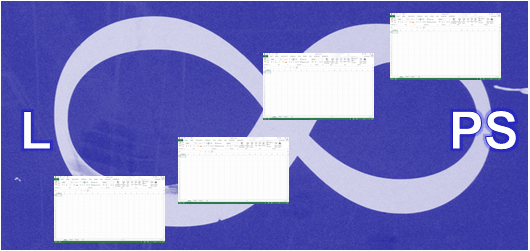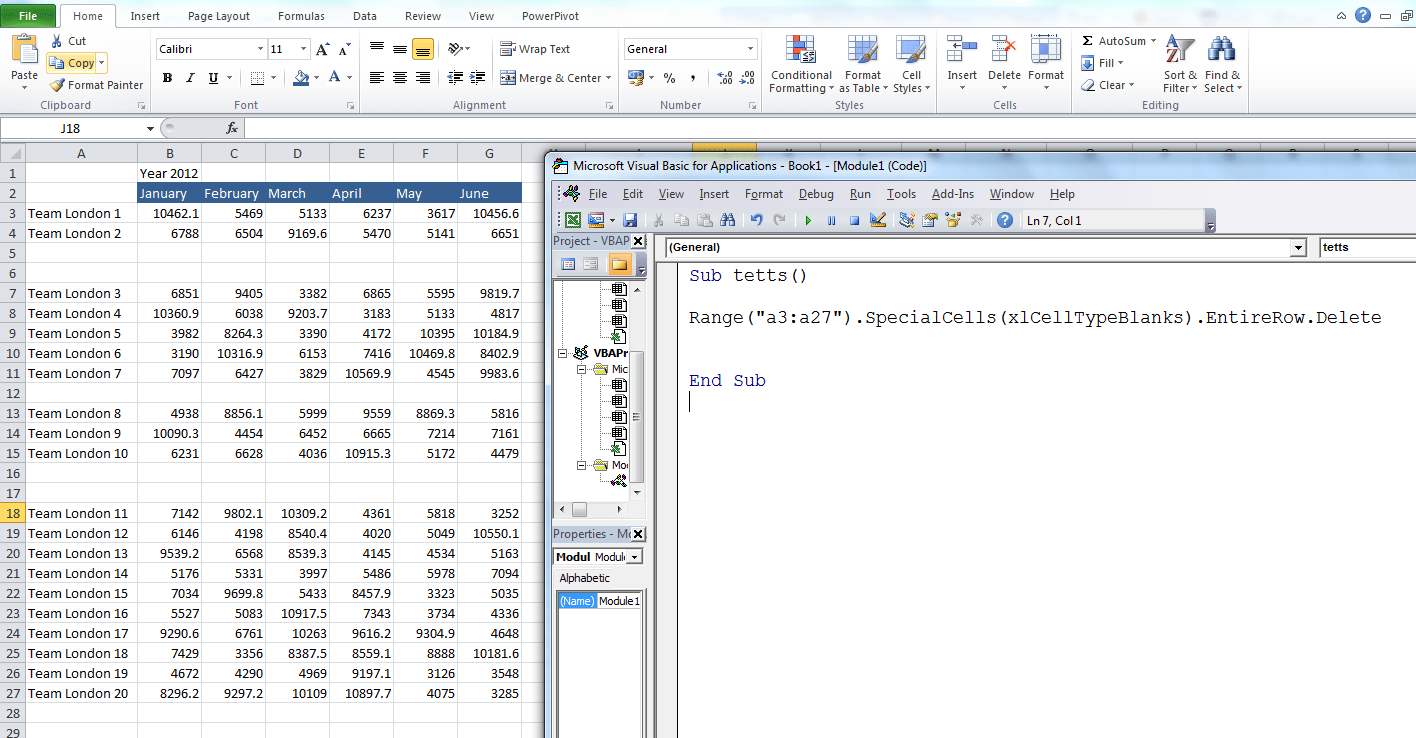## Using loops to easily make changes across multiple worksheets

Loops are one of the key tools in Excel VBA when we need to perform tasks through a number of objects (cells, worksheets, charts, workbooks etc.) .  Here we will look at how to loop through Excel worksheets and workbooks.

## Loop Through Excel WorksheetsBelow you will find three examples using different loops but all three will perform exactly the same task.

## The For Each loop

1. An object variable (sh) is used and declared as Worksheet to tell Excel that we want store worksheets (the address) in the memory  of our computer (Dim sh As Worksheet).
2. The For Each loop will loop through each worksheet in the active workbook (For Each sh In ActiveWorkbook.Sheets).
3. The code will add 500 in A1 in all sheets in the active workbook.

Sub LoopSheets()
Dim sh As Worksheet
For Each sh In ActiveWorkbook.Sheets
sh.Range(“A1”).Value = 500
Next sh
End Sub

## The For Next loop

1. A data variable is used to store a whole number (integer) in the computer’s memory (Dim iCounter As Integer).
2. The For Next loop is used to loop through all sheets in the active workbook but the loop needs to know how many worksheets there is in the active workbook (ActiveWorkbook.Sheets.Count).
3. The iCounter variable is used to move through the worksheets and the value 500 is entered in A1 in all worksheets in the active workbook (Sheets(iCounter).Range(“A1”).Value = 500).

Sub LoopSheetst2()
Dim iCounter As Integer
For iCounter = 1 To ActiveWorkbook.Sheets.Count
Sheets(iCounter).Range(“A1”).Value = 500
Next iCounter
End Sub

## The Do loop

1. A data variable is used to store a whole number (integer) in the computer’s memory (Dim iCounter As Integer).
2. 1 is stored in the iCounter variable (iCounter = 1).
3. Do Until loop is used to run until criteria is met in this example until the value in the variable iCounter is total number of worksheets in the active workbook plus one (Do Until iCounter = ActiveWorkbook.Worksheets.Count + 1).

Sub LoopSheets3()
Dim iCounter As Integer
iCounter = 1
Do Until iCounter = ActiveWorkbook.Worksheets.Count + 1
Sheets(iCounter).Range(“A!”).Value = 500
iCounter = iCounter + 1
Loop
End Sub

## Loop WorkbooksBelow you will find three examples using different loops but all three will perform exactly the same task but this time the loops will loop through workbooks.

## The For Each loop

1. An object variable (wBook) is used and declared as Workbook to tell Excel that we want store workbooks (the address) in the memory of our computer (Dim WBook As Workbook).
2. The For Each loop will loop through each open workbook  (For Each wBook In Workbooks).
3. The code will add 2 in A2 in sheet 1 in all open workbooks.

Sub LoopWorkBooks()
Dim WBook As Workbook
For Each WBook In Workbooks
WBook.Sheets(1).Range(“A2”).Value = 2
Next WBook
End Sub

## The For Next loop

1. A data variable is used to store a whole number (integer) in the computer’s memory (Dim iWB As Integer).
2. The For Next loop is used to loop through all open workbooks but the loop needs to know how many open workbooks we have (Workbooks.Count).
3. The iWB variable is used to move through the open workbooks and the value 2 is entered in A2 in sheet 1 in all open workbooks (Workbooks(iWB).Sheets(1).Range(“A2”).Value = 2).

Sub LoopWorkBooks2()
Dim iWB As Integer
For iWB = 1 To Workbooks.Count
Workbooks(iWB).Sheets(1).Range(“A2”).Value = 2
Next iWB
End Sub

## The Do loop

1. A data variable is used to store a whole number (integer) in the computer’s memory (Dim iCounter As Integer).
2. 1 is stored in the iCounter variable (iCounter = 1).
3. Do Until loop is used to run until the criteria is met in this example until the value in the variable iCounter is total number of open workbooks plus one (Do Until iCounter = Workbooks.Count + 1).

Sub LoopWorkBooks3()
Dim iCounter As Integer
iCounter = 1
Do Until iCounter = Workbooks.Count + 1
Workbooks(iCounter).Sheets(1).Range(“A2”).Value = 2
iCounter = iCounter + 1
Loop
End Sub

## Loop workbooks & worksheetsx

In the examples below nested loops are looping through workbooks and worksheets and again the For EachFor Next and the Do loop are used to do the job.

## The For Each loop

Exactly as in the examples above in this post variables are used to store the address of the workbooks and worksheets in the computers memory (Dim WBook As Workbook & Dim sh As Worksheet). A For Each loop is used to run through the workbooks and one to run through the worksheets.

Sub LoopWorkBookSheets()
Dim WBook As Workbook
Dim sh As Worksheet
For Each WBook In Workbooks
For Each sh In WBook.Worksheets
sh.Range(“a1”) = 2
Next sh
Next WBook
End Sub

## The For Next loop

Two For Next loops are needed to run through all worksheets in all open workbooks. Two variables are used (counter variables) to loop one workbook at the time and one worksheet.

Sub LoopWorkBookSheets2()
Dim iWB As Integer
Dim iCounter As Integer
For iWB = 1 To Workbooks.Count
For iCounter = 1 To Workbooks(iWB).Sheets.Count
Workbooks(iWB).Sheets(iCounter).Range(“b1”).Value = 450
Next iCounter
Next iWB
End Sub

## The Do loop

It takes more coding to run through all worksheets in all open workbooks by using the Do loop. Again two loops are needed one for the workbooks and one for the worksheets.

Sub LoopWorkBookSheets3()
Dim iWorkBookCounter As Integer
Dim iSheetCounter As Integer
iWorkBookCounter = 1
iSheetCounter = 1
Do Until iWorkBookCounter = Workbooks.Count + 1
Do Until iSheetCounter = Workbooks(iWorkBookCounter).Sheets.Count + 1
Workbooks(iWorkBookCounter).Sheets(iSheetCounter).Range(“c1”).Value = 5
iSheetCounter = iSheetCounter + 1
Loop
iWorkBookCounter = iWorkBookCounter + 1
iSheetCounter = 1
Loop
End Sub

Some people prefer to use the For Each loop for a couple of reasons. The For Each loop is a faster loop and normally you need less coding.

Excel course is one of our Microsoft Office 365 training courses.

### Useful Resources

Macro to Loop Through All Worksheets in a Workbook

Excel VBA 2013: Track Changes With the Inquire Add-In

## How to use SpecialCells method in Excel VBA

SpecialCells in VBA is a really useful method to deploy in Excel. It returns a Range Object that only covers the type of cells you specify. You can use the SpecialCells in VBA Method to return a Range Object that only holds numbers, text, blank cells, formulae, cells with datavalidation, cells with conditional formatting, the last cell in the worksheet, cells with comments and all visible cells.

If you for example want to change formatting for all numbers in a worksheet you do not need more than one line in the Visual Basic Editor to do it.

Cells.SpecialCells(xlCellTypeConstants, xlNumbers).Style = “currency”

This line will change all numbers in the active worksheet to currency format.  The Range object Cells is used to tell Excel that you want to look at all the cells and the special cells method to decrease it to in this example only constants (xlCellTypeConstants) and again to decrease it to only numbers the criteria  xlNumbers is added to the SpecialCells Method.

Similar we can use the SpecialCells Method to return a Range Object that only holds text.

Cells.SpecialCells(xlCellTypeConstants, xlTextValues).Font.ColorIndex=3

This VBA line will change the font colour to red for all text in the active worksheet.

The SpecialCells Method syntax is;
expression.SpecialCells(Type, Value)

The Expression have to be a Range object such as Cells, Range(“A1:B200”), ActiveSheet.UsedRange etc.

The different types of special cells are:

1. xlCellTypeAllFormatConditions (all formatted cells)
2. xlCellTypeAllValidation (all cells with datavalidation)
3. xlCellTypeBlanks (all blank cells)
4. xlCellTypeComments (all cells with notes)
5. xlCellTypeConstants (all cells containing constants (numbers or text))
6. xlCellTypeFormulas (all cells with formulas)
7. xlCellTypeLastCell (The last cell in all used ranges)
8. xlCellTypeSameFormatConditions (all cells with the same formatting also conditional formatting)
9. xlCellTypeSameValidation (all  cells with the same datavalidation)
10. xlCellTypeVisible (alll visible cells)

You can also use a combination of the above options.

Cells.SpecialCells(xlCellTypeConstants, xlNumbers).SpecialCells(xlCellTypeAllValidation).Font.Color = vbRed

This line of VBA code will add red font colour to all cells with numbers & Datavalidation.

The SpecialCells in VBA Method is also very powerful if you want to test your data in an If Then Else decision code.

In the example above all numbers are tested in the active worksheet if the value is greater than 7500. If the test is true 10% is added. The For Each loop is only running through cells with numbers.

The SpecialCells in VBA Method can be very handy if you need to remove blank rows from you Excel lists or Excel databases.and after running the macroIn the above example The SpecialCells Method finds all blank cells in the range from A3 to A27 and deletes the entire row.

You have a lot of variations you can use and you will find out that when you start using The SpecialCells method  you will save a lot of lines in your macros!